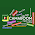## 10 -10 × 10 + 10=?: what is the answer

This puzzle and many others are trending on internet today. The obvious reasons is simply the deadly Corona virus and it divastating effects in the world.

Due to the deadly Corona virus, many countries around the world are on total or partial lockdown in bit to reduce the spread of virus.

Because of this, many People isolate themselves and fill bored. Hence, engaging online with family and friends playing games, solving quzzes, riddles, puzzles and more.

No doubt we recenly had a spike in number of people visiting our site in search of solutions to puzzles and Quizes.

Math puzzle and Quizes may simple to those familiar with mathematics but tuffer to those who are not familiar with math. This is why we deemed it nessecory to solve it step by step with explainations.

Solving math puzzles can refresh your memory and make you to be able to judge things logically.

You may also like to read our trending puzzle: Chicken, egg and banana Puzzle answer.

Here's is the puzzle for today:
If
😷 + 😷 + 😷=60,

🙎‍♂️+🙎‍♂️- 😷 = 💀,

💀= -4,
Then

💀+ 😷 × 🙎= ?
See screen below.Face mask Imoji, Man and skeleton puzzle

Solution:
Let's solve each equation separately.

Equation one:
Face mask Imoji = 60 ÷ 3,
Implies face mask Imoji = 20

Equation Two.
Man + Man - face mask Imoji = skeleton.
The value of face mask is 10 and that of skeleton is - 4. So, we substitute these values and solve as thus:
2men - 20 = - 4,
2men = - 4 + 20
2men = 16.
Man = 16÷2

Man = 8

Final equation:
Man = 8
Skeleton = - 4.
Let's substitute these values in the final equation.
- 4 +20 × 8= ?
Using BODMAS,

-4 + 160 = ?
156= ?,

The final answer to the puzzle is 156.

Recommended for you:

1.Shoes man and paper puzzle answer.

### Mbah Derick A.

I am a Banker by profession and a writer by occupation. I like teaching, learning and collaborating with others.💚 Please share my post!

1.I'm really lost on this. Why is suddenly the face mask emoji 10 in the second part? We established that equals 20 above. So 2x-20=-4, hence x (being man) = 8.

1.This comment has been removed by the author.

2.Hi it's and error and has been corrected. Thanks